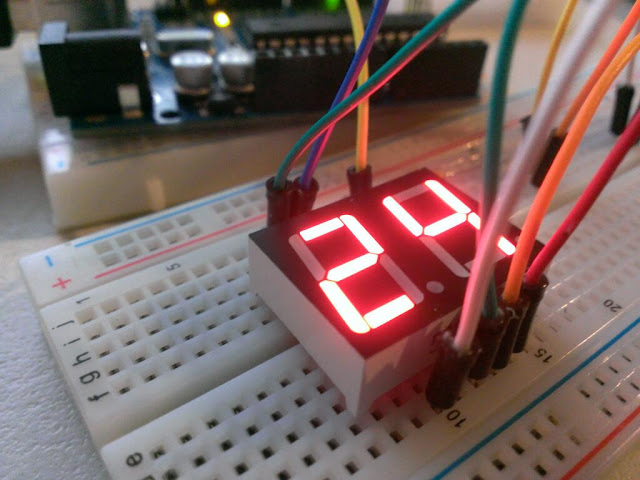# 7 Segment 2 Digit and 10 pins Counter 00-99 with Arduino UNO

Today we are going to create a  project with 7-segment LED 2-digit by using Arduino UNO, include the wiring and coding.  Now to see this project step by step:Figure 1: 7-Segment 2-digit 10-pins

### Project Requirement:

• Arduino UNO
• Jump Wire
• 7-Segment 2-Digit 10-pins LED

### 1. Understand the segments in 7-Segment LED

7-Segment LED has 7 segments LED to display the number from Zero to Nine for each one digit. Each segment has is own variable and work independently. See the figure 2 below:Figure 2: 7 Segments and Binary Code

To set the 7-Segment LED with binary code, you have to write the code sequence from a-g and left to right with start up binary header "0b".Figure 3.7-segment 2-digit 10-pins

Figure 3 show the 7-segment 2-digit 10-pins, how to set each pin on this LED.

a, b, c, d, e, f and g are not on order, so please carefully in wiring. Otherwise your LED display will not show the number you want.

"ds1" is the power pin to support digit 1 on the right side and "ds2" is the power pin to support digit 2 on the left side. "dp" is point pin next to the digit buttom.

Make sure you have connected with the right pin of Arduino and 7-Segment LED.

### 2. Circuit Wiring

Set Arduino UNO digital pin from 2 to 8 are the pins to control the 7-Segment display. Arduino UNO pin 12 & 13 are the switch power for the two digits on 7-Segment LED.Figure 4. Arduino UNO and 7-Segment Wiring

### 3. Coding

1. int digit = {0b0111111, 0b0000110, 0b1011011, 0b1001111, 0b1100110, 0b1101101, 0b1111101, 0b0000111, 0b11111111, 0b1101111};
2.
3. int digit1, digit2;
4.
5. void setup()
6. {
7.   for (int i = 2; i < 9; i++)
8.   {
9.     pinMode(i, OUTPUT);
10.   }
11.   pinMode(12, OUTPUT);
12.   pinMode(13, OUTPUT);
13. }
14.
15. void loop() {
16.   for (int j = 0; j <= 99; j++)
17.   {
18.     digit2 = j / 10;
19.     digit1 = j % 10;
20.   for ( int k = 0; k < 20; k++)
21.   {
22.       digitalWrite(12, HIGH);
23.       digitalWrite(13, LOW);
24.       dis(digit2);
25.       delay(10);
26.
27.       digitalWrite(13, HIGH);
28.       digitalWrite(12, LOW);
29.       dis(digit1);
30.       delay(10);
31.   }
32.   }
33. }
34.
35. void dis(int num)
36. {
37.   for (int i = 2; i < 9; i++)
38.   {
39.     digitalWrite(i, bitRead(digit[num], i - 2));
40.   }
41. }

### Test the Project

You will counter from 00 to 99 and loop this count on you 7-Segment LED.Figure 5. Testing
Now let enjoy you day guys, leave you comment below this post for you idea in this project.

Thank!!!